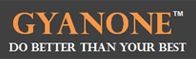# GMAT Simple interest

One of the topics commonly tested in the GMAT Quant section is that of Simple Interest. While the formulas and concepts applied herein are simple enough, a lot of test takers get caught up in the terminology surrounding the concepts and therefore get their answers on this topic wrong. Here, then, are the concepts on Simple Interest that you can get tested on when you take the GMAT, including the terminology:

Simple Interest = P x R x T/100

Where P: Principal (or the amount originally lent out)
R: Rate of interest (expressed in terms of %)
T: Time period for which the loan is made

Common mistakes made by test takers when dealing with Simple Interest problems

1. Taking the rate of interest in decimals, instead of in percentage terms: Remember, the 100 in the denominator of the formula is there because it assumes that the rate of interest (R) will be taken in percentage terms, not in decimals.

Example: If P = \$1000, R = 4% per annum, and T = 3 years,
SI = 1000 x 4 x 3 / 100 = 120

Do NOT take SI = 1000 x 0.04 x 3 / 100 = 1.2

2. Interpreting SI to mean the amount to be paid back: The SI formula calculates just the interest to be paid back at the end of the loan period. It does NOT include the amount.

Example: If \$1000 is loaned for 3 years at 4% annual rate of interest, the amount to be paid back at the end of the 3 years = 1000 x 3 x 4 /100 + 1000 = 1120

Remember to add the principal when calculating the amount due.

3. Adjusting both the time and the rate of interest when dealing with periods of loan that are not full years: Remember, if the calculation is to be made on a semi-annual basis, then adjust either the time period or the rate of interest, not both.

Example: If \$1000 is loaned for 6 months at 4% annual rate of interest then
SI = 1000 x 4 x (6/12)/100 = 20 [Dividing the time period by 12 to convert it into half an year or 6 months]

OR

SI = 1000 x (4/2) x 6 /100 = 20 [Dividing the annual rate of interest by 2 to convert it into a monthly rate of interest]

DO NOT make the mistake of using the formula as:

SI = 1000 x (4/2) x (6/12) / 100 = 10 [Here the rate of interest has been converted to a monthly rate but the time period has been converted from months (6) to years (6/12), which IS WRONG]

Author :GyanOne is an educational services firm focused on premium GMAT coaching, application advisory (with a focus on ISB applications), and interview preparation for B-school admissions. GyanOne operates in the New Delhi region of India and has top global B-school alumni with a minimum score of 770 on the GMAT, as instructors. All GyanOne counsellors are professionally trained top B-school alumni with an experience of 100+ MBA applications behind them.1. Complete GMAT RC Questions in less than 1 minute and 50 seconds
3. Take Notes Effectively
4. Collect and Interpret Facts
5. Speed up Summary Creation
6. Remember Information
7. Question the Author

12. Learn to Answer GMAT organization of passage Question
13. Learn to identify the style/tone or attitude of the author

Mastering GMAT Critical ReasoningAfter you read F1GMAT’s Mastering GMAT Critical Reasoning Guide, you will learn:

How to overcome flawed thinking in GMAT Critical Reasoning?

How to spot Inconsistencies in Arguments

How to eliminate out of scope answer choices using Necessary and Sufficient Conditions

How to Paraphrase GMAT Critical Reasoning Question

How to Answer Assumption Question Type

How to Answer Conclusion Question Type

How to Answer Inference Question Type

How to Answer Strengthen Question Type

How to Answer Weaken Question Type

How to Answer bold-faced and Summary Question Types

How to Answer Parallel Reasoning Questions

How to Answer the Fill in the Blanks Question

Get F1GMAT's Newsletters (Best in the Industry)

• Ranking Analysis
• Post-MBA Salary Trends
• Post-MBA Job Function & Industry Analysis
• Post-MBA City Review
• MBA Application Essay Tips
• School Specific Essay Tips
• GMAT Preparation Tips